• Java基础语法(上) 一.关键字&标识符 提示：以下是本篇文章正文内容，下面案例可供参考 1.关键字 ​ Java关键字是电脑语言里事先定义的，有特别意义的标识符，有时又叫保留字，还有特别意义的变量。Java的关键字...
Java基础语法(上)
文章目录Java基础语法(上)一.关键字&标识符1.关键字2.标识符2.1各种类型命名规范二.Java数据类型1.基本数据类型基本数据类型之间的转换2.引用数据类型基本类型与引用类型的区别三.运算符1.算数运算符+++ & --2.比较运算符3.逻辑运算符4.赋值运算符5.条件运算符6.位运算符
一.关键字&标识符
提示：以下是本篇文章正文内容，下面案例可供参考
1.关键字
​		Java关键字是电脑语言里事先定义的，有特别意义的标识符，有时又叫保留字，还有特别意义的变量。Java的关键字对Java的编译器 有特殊的意义，他们用来表示一种数据类型，或者表示程序的结构等，关键字不能用作变量名、方法名、类名、包名和参数。
书写时必须注意关键字为小写,Java中严格区分大小写
关键字按照作用不同可分为:

访问控制

类方法变量修饰符

程序控制

错误处理

包相关

基本类型

变量引用

保留字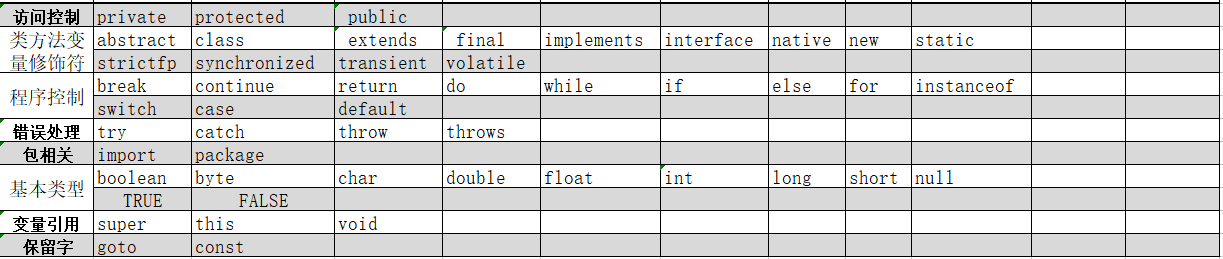图片来源: https://blog.csdn.net/z1web/article/details/89068217

下面是Java中每个关键字的含义

关键字
含义

abstract
表明类或者成员方法具有抽象属性

assert
断言，用来进行程序调试

boolean
基本数据类型之一，声明布尔类型的关键字

break
提前跳出一个块

byte
基本数据类型之一，字节类型

case
用在switch语句之中，表示其中的一个分支

catch
用在异常处理中，用来捕捉异常

char
基本数据类型之一，字符类型

class
声明一个类

const
保留关键字，没有具体含义

continue
回到一个块的开始处

default
默认，例如，用在switch语句中，表明一个默认的分支。Java8 中也作用于声明接口函数的默认实现

do
用在do-while循环结构中

double
基本数据类型之一，双精度浮点数类型

else
用在条件语句中，表明当条件不成立时的分支

enum
枚举

extends
表明一个类型是另一个类型的子类型。对于类，可以是另一个类或者抽象类；对于接口，可以是另一个接口

final
用来说明最终属性，表明一个类不能派生出子类，或者成员方法不能被覆盖，或者成员域的值不能被改变，用来定义常量

finally
用于处理异常情况，用来声明一个基本肯定会被执行到的语句块

float
基本数据类型之一，单精度浮点数类型

for
一种循环结构的引导词

goto
保留关键字，没有具体含义

if
条件语句的引导词

implements
表明一个类实现了给定的接口

import
表明要访问指定的类或包

instanceof
用来测试一个对象是否是指定类型的实例对象

int
基本数据类型之一，整数类型

interface
接口

long
基本数据类型之一，长整数类型

native
用来声明一个方法是由与计算机相关的语言（如C/C++/FORTRAN语言）实现的

new
用来创建新实例对象

package
包

private
一种访问控制方式：私用模式

protected
一种访问控制方式：保护模式

public
一种访问控制方式：共用模式

return
从成员方法中返回数据

short
基本数据类型之一,短整数类型

static
表明具有静态属性

strictfp
用来声明FP_strict（单精度或双精度浮点数）表达式遵循[IEEE 754](https://baike.baidu.com/item/IEEE 754)算术规范

super
表明当前对象的父类型的引用或者父类型的构造方法

switch
分支语句结构的引导词

synchronized
表明一段代码需要同步执行

this
指向当前实例对象的引用

throw
抛出一个异常

throws
声明在当前定义的成员方法中所有需要抛出的异常

transient
声明不用序列化的成员域

try
尝试一个可能抛出异常的程序块

void
声明当前成员方法没有返回值

volatile
表明两个或者多个变量必须同步地发生变化

while
用在循环结构中

注意:null,false,true这几个保留字不是关键字,而是文字,包含Java定义的值.不可当标识符使用
2.标识符
标识符分为关键字和用户自定义标识符
对类,类的对象,方法,变量等要素进行的定义命名,标识符可有一个或多个字符.在Java中,其命名规则如下

标识符由数字（0~9）、美元符号（$）、下划线（_）以及 Unicode 字符集中符号大于 0xC0 的所有符号组合构成. 必须以字母、下划线和美元符号开头，后面可以是任何字母、数字、美元符号或下划线. 不能以数字开头，也不能使用任何 Java 关键字作为标识符 . 标识符可以包含关键字，但不能与关键字重名. 严格区分大小写,不能有空格. 不能使用中文及拼音 2.1各种类型命名规范 项目名全部小写 (project) 包名全部小写 (package) 类名首字母大写,其余组成词首字母依次大写. (CarClass) 变量名,方法名首字母小写,如果名称由多个单词组成,除首字母外的每个单词的首字母都要大写. (newTest) 常量名全部大写. (MAX) 二.Java数据类型 1.基本数据类型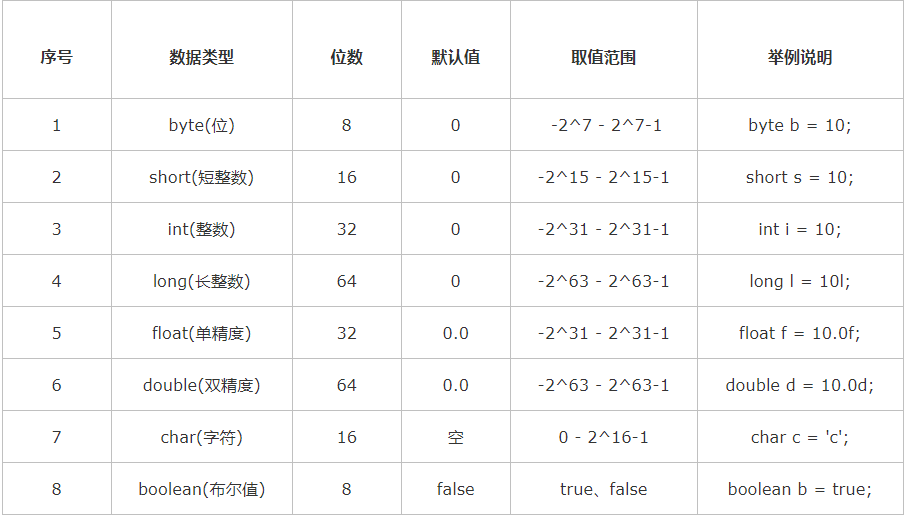其中声明long型与float型变量时,需添加后缀(l或L,F或f)上图,idea未报错不加就会报错 基本数据类型之间的转换 默认转换 小容量默认转换为大容量 容量从小到大依次为: byte/short/char–>int–>long–>float–>double byte,short,char三者在运算中会转换为int,而不会相互转换 操作数1类型 操作数2 类型 转换后的类型 byte、short、char int int byte、short、char、int long long byte、short、char、int、long float float byte、short、char、int、long、float double double 强制转换 隐式强制转换 在进行算数操作时，会把结果的类型“隐式”提升为 int 类型，此时再使用 short/byte/char 类型的变量引用时，就会出现“损失精度” short s=2; byte b=3; char c='a'; //隐式强制转换 short s1=s+b+c; byte b1=s+b+c; char c1=s+b+c;  运行结果:解决方法: 此時可以用int 或字节更大的类型变量来接收运算结果 对运算结果进行强制转换 short s=2; byte b=3; char c='a'; //用字节大的来接收,或强制转换 short s2=(short)(s-b+c); int i=s-b+c;  显式强制转换 //显式强制转换 int m; double d=10.51; m=(int)d; System.out.println("m="+m); System.out.println("d="+d);  结果:2.引用数据类型 引用数据类型: 类、 接口类型、 数组类型、 枚举类型、 注解类型、 字符串型 所有非基本数据类型都可称为引用数据类型 基本类型与引用类型的区别 基本变量类型 在方法中定义的非全局基本数据类型变量的具体内容是存储在栈中的 引用变量类型 只要是引用数据类型变量，其具体内容都是存放在堆中的，而栈中存放的是其具体内容所在内存的地址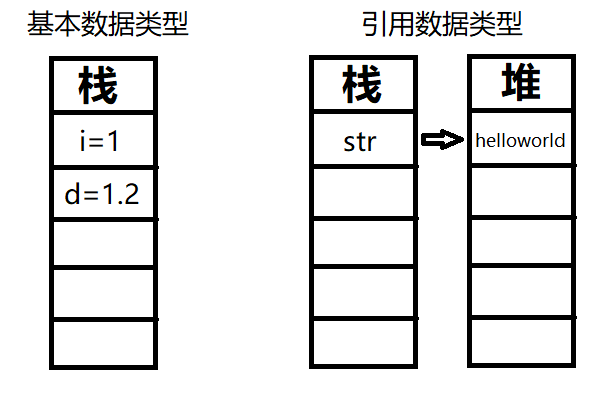//基本数据类型 int a=100; double d=1000.0; boolean b=false; //引用数据类型 Scanner sc=new Scanner(System.in); String str=sc.nextLine();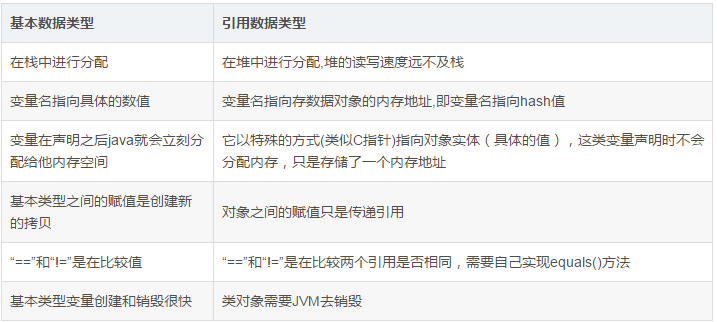三.运算符 1.算数运算符 运算符 功能 + 1.算数运算 2.连接字符串 - 减 * 乘 / 求商(取整) ^ 求余/模 ++ 自增 – 自减 + int a=10; short b=10; String str="abc"; System.out.println(a+b);//算数运算 /* 若 "+"后紧跟字符串,则字符串后的所有"+"都为字符串连接符 字符串前的"+"为算数运算符 */ System.out.println(str+a+b); System.out.println(b+a+str+b+a); System.out.println(a+b+str+b);  运算结果:注意: ​ 若 "+"后紧跟字符串,则字符串后的所有"+"都为字符串连接符,将后面的数值转换为字符串进行连接 字符串前的"+"为算数运算符,数值也依旧按照规则运算后再和字符串连接 ++ & – ++: int a=0; int b=a++; //先赋值(0),再自增 a=1,b=0; int c=++a; //先自增(a++-->a=a+1-->a=1+1=2),再赋值(c=2) System.out.println("b="+b); System.out.println("c="+c);  运算结果:- - 与++同理. 2.比较运算符 运算符 运算 范例 结果 == 相等于 4==3 false != 不等于 4!=3 true < 小于 4<3 false > 大于 4>3 true <= 小于等于 4<=3 false >= 大于等于 4>=3 true instanceof 检查是否是类的对象 “Hello” instanceof String true 比较运算符的结果都是boolean型(false/true). 3.逻辑运算符  逻辑运算符要求操作数的数据类型为逻辑型，其运算结果也是逻辑型值。  ​ 逻辑运算符的真值表 操作数1 操作数2 短路与 短路或 逻辑非 逻辑异或 逻辑与 逻辑或 A B A && B A || B ! A A ∧ B A & B A | B T T T T F F T T T F F T F T F T F T F T T T F T F F F F T F F F 其中 & | ^ ! 既可参与逻辑运算(false,true) 也可参与位运算(二进制bit位运算 ,eg: 5^10) 逻辑与 | : 左边无论真假，右边都进行运算 短路或 ||: 遇到第一个true发生短路,不再执行||后的语句 逻辑或 & : 左边无论真假，右边都进行运算 短路与 &&: 遇到第一个false发生短路,不再执行&&后的语句 逻辑异或 ^ : 当左右都为true时，结果为false "异"时为真,"同"时为假 4.赋值运算符 运算符 意义 实例 等价于 = 赋值 n=25 += 加赋值 n += 25 n=n+25 -= 减赋值 n -= 25 n=n-25 *= 乘赋值 n *= 25 n=n*25 /= 除赋值 n /= 25 n=n/25 %= 求余赋值 n %= 25 n=n%25 <<= 左移位赋值 n <<= 25 n=n<<25 >>= 右移位赋值 n >>= 25 n=n>>25 &= 按位与赋值 n &= OxF2F2 n=n&OxF2F2 ^= 按位异或赋值 n ^= OxF2F2 n=n ^ OxF2F2 |= 按位或赋值 n |= OxF2F2 n=n | OxF2F2 5.条件运算符 条件运算符优先级高于赋值、逗号运算符，低于其他运算符。 运算符: 条件表达式?(表达式1):(表达式2); 当条件表达式的结果 为true时整个条件表达式结果是表达式1的值 为false时整个条件表达式结果是表达式2 的值 eg. max=(a>b)?a:b 将a和b二者中较大的一个赋给max。 当一个表达式中出现多个条件运算符时，应该将位于最右边的问号与离它最近的冒号配对，并按这一原则正确区分各条件运算符的运算对象。  eg. w < x ? x + w : x < y ? x : y ​ 与 w < x ? x + w : ( x < y ? x : y) 等价 ​ 与 (w < x ? x + w : x < y) ? x : y 不等价 ​ a > b ? c > d ? e : f : g ​ 与a > b ? (c > d ? e : f) : g等价 6.位运算符 Operator Usage Description 按位与 a & b 在a,b的位表示中，每一个对应的位都为1则返回1， 否则返回0. 按位或 a | b 在a,b的位表示中，每一个对应的位，只要有一个为1则返回1， 否则返回0. 按位异或 a ^ b 在a,b的位表示中，每一个对应的位，两个不相同则返回1，相同则返回0. 按位非 ~ a 反转被操作数的位。 左移 a << b 将a的二进制串向左移动b位,右边移入0. 算术右移 a >> b 把a的二进制表示向右移动b位，丢弃被移出的所有位 (算术右移左边空出的位是根据最高位是0和1来进行填充的) 无符号右移(左边空出位用0填充) a >>> b 把a的二进制表示向右移动b位，丢弃被移出的所有位，并把左边空出的位都填充为0 package p1.OperatorDemo; import java.util.Scanner; /* * 位运算符 * 按位与 & 1&1=1 1&0=0 0&1=0 * 按位或 | 1|1=1 1|0=1 0|1=1 * 按位异或 ^ 1^1=0 0^0=0 1^0=1 0^1=1 * 按位取反 ~ 二进制按补码各位取反 * 左移 << * (带符号)右移 >> * 无符号右移 >>> * */ public class C1_5 { public static void main(String[] args){ Scanner sc=new Scanner(System.in); System.out.println("输入两个数,得其按位与/或/异或/取反的结果"); int a=sc.nextInt(); int b=sc.nextInt(); // & | ~ ^ // 0010 // 0011 System.out.println("按位与: "+(a&b));// 0010 System.out.println("按位或: "+(a|b));// 0011 System.out.println("按位异或: "+(a^b));// 0001 System.out.println("按位取反: "+(~++a));// System.out.println("按位取反: "+(~b));// System.out.println(); System.out.println("输入一个数,得其左右移运算结果:\r\n"); int c=sc.nextInt(); System.out.println("你想左/右移多少位?"); int x=sc.nextInt(); System.out.println(); // 左移 一位 相当于乘2的1次方 System.out.println("左移: "+(c<<x)); // (带符号)右移 一位 相当于除2的1次方 System.out.println("带符号右移: "+(c>>x)); //无符号右移 System.out.println("无符号右移:"+(a>>>x)); } }  运行结果:本人新✋,初来乍到,:✒️此拙作以记录学习中的知识点滴,若能帮助到同在学习的新手,不甚荣幸.若有不对的地方,还请各位大佬斧正,不吝赐教🙏!  展开全文• 特点：关键字所有字母都小写 有以下几种类型的关键字： 保留字 现有的Java版本尚未使用，但以后可能会作为关键字使用。例如：const、goto 二、标识符 定义：Java 对各种变量、方法和类等... 一、关键字与保留字 关键字 定义：被Java语言赋予了特殊的含义，用作专门用途的字符串 特点：关键字所有字母都为小写 有以下几种类型的关键字：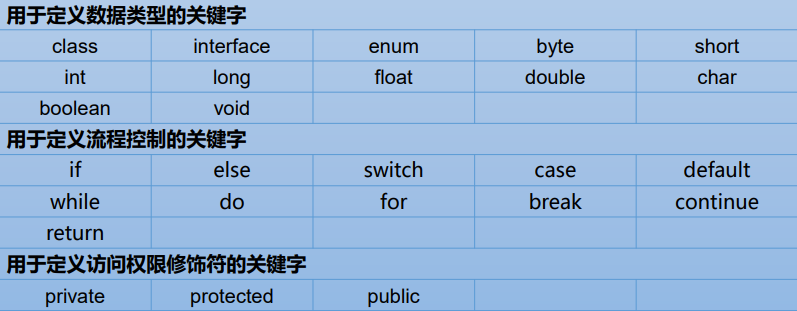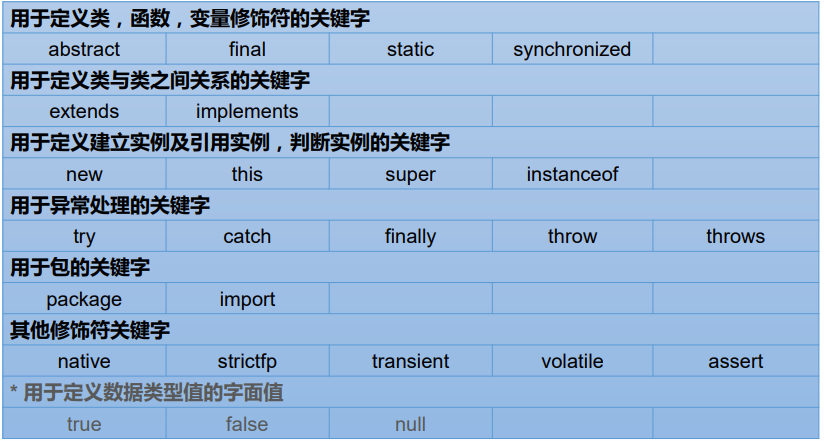保留字 现有的Java版本尚未使用，但以后可能会作为关键字使用。例如：const、goto 二、标识符 定义：Java 对各种变量、方法和类等要素命名时使用的字符序列称为标识符 技巧：凡是自己可以起名字的地方都叫标识符。 规则： 由26个英文字母大小写，0-9 ，_或$ 组成
数字不可以开头。
不可以使用关键字和保留字，但能包含关键字和保留字。
Java中严格区分大小写，长度无限制。
标识符不能包含空格。

命名规范

包名：多单词组成时所有字母都小写：xxxyyyzzz

类名、接口名：多单词组成时，所有单词的首字母大写：XxxYyyZzz

变量名、方法名：多单词组成时，第一个单词首字母小写，第二个单词开始每个 单词首字母大写：xxxYyyZzz

常量名：所有字母都大写。多单词时每个单词用下划线连接：XXX_YYY_ZZZ
注意1：在起名字时，为了提高阅读性，要尽量有意义，“见名知意”
注意2：java采用unicode字符集，因此标识符也可以使用汉字声明，但是不建议使用

三、变量
1、变量的概念：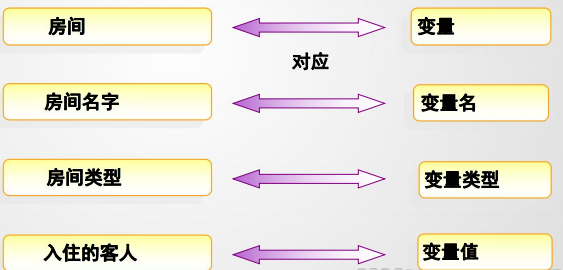内存中的一个存储区域
该区域的数据可以在同一类型范围内不断变化
变量是程序中最基本的存储单元。包含变量类型、变量名和存储的值

内存中变量好比旅馆的房间，内存中常量好比住旅馆房间的人
2、变量的作用： 用于在内存中保存数据
3、使用变量注意：

Java中每个变量必须先声明，后使用
使用变量名来访问这块区域的数据
变量的作用域：其定义所在的一对{ }内
变量只有在其作用域内才有效
同一个作用域内，不能定义重名的变量

4、声明变量
语法：<数据类型> <变量名称>
例如：int var;
5、变量的赋值
语法：<变量名称> = <值>
例如：var = 10;
6、声明和赋值变量
语法： <数据类型> <变量名> = <初始化值>
例如：int var = 10;
7、变量的分类
按数据类型
对于每一种数据都定义了明确的具体数据类型（强类型语言），在内存中分 配了不同大小的内存空间。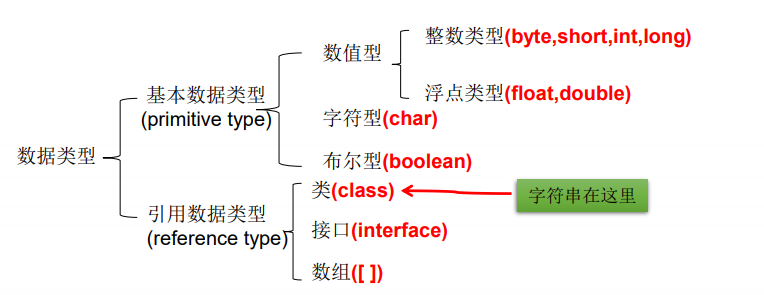按声明的位置的不同
在方法体外，类体内声明的变量称为成员变量。
在方法体内部声明的变量称为局部变量。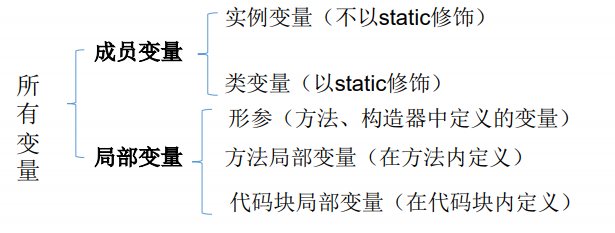四、基本数据类型
1.整数类型：byte、short、int、long

Java各整数类型有固定的表数范围和字段长度，不受具体OS的影响，以保 证java程序的可移植性。
java的整型常量默认为 int 型，声明long型常量须后加‘l’或‘L’
java程序中变量通常声明为int型，除非不足以表示较大的数，才使用long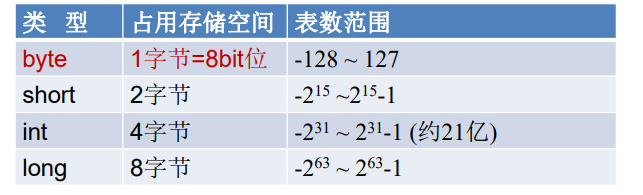2.浮点类型：float、double

与整数类型类似，Java 浮点类型也有固定的表数范围和字段长度，不受具体操作 系统的影响。 浮点型常量有两种表示形式：

十进制数形式：如：5.12 512.0f .512 (必须有小数点）
科学计数法形式:如：5.12e2 512E2 100E-2

float:单精度，尾数可以精确到7位有效数字。很多情况下，精度很难满足需求。

double:双精度，精度是float的两倍。通常采用此类型。

Java 的浮点型常量默认为double型，声明float型常量，须后加‘f’或‘F’。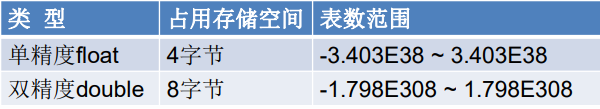浮点数不适用于禁止出现舍入误差的金融计算中
例如，命令System.out.println(2.0-1.1);将打印出0.8999999999999999，而不是人们希望的0.9。出现这种问题的主要原因在于浮点数值是采用二进制系统表示的。而在二进制系统中无法精确的表示分数1/10，这就好像十进制无法精确表示1/3一样。
3.字符类型：char

char 型数据用来表示通常意义上“字符”(2字节)
Java中的所有字符都使用Unicode编码，故一个字符可以存储一个字母，一个汉字，或其他书面语的一个字符。
字符型变量的三种表现形式：

字符常量是用单引号(‘ ’)括起来的单个字符。例如：char c1 = ‘a’; char c2 = ‘中’; char c3 = ‘9’;
Java中还允许使用转义字符‘\’来将其后的字符转变为特殊字符型常量。 例如：char c3 = ‘\n’; // '\n’表示换行符
直接使用 Unicode 值来表示字符型常量：‘\uXXXX’。其中，XXXX代表 一个十六进制整数。如：\u000a 表示 \n。

char类型是可以进行运算的。因为它都对应有Unicode码。
4.布尔类型：boolean

boolean 类型用来判断逻辑条件，一般用于程序流程控制：

if条件控制语句；
while循环控制语句；
do-while循环控制语句；
for循环控制语句；

boolean类型数据只允许取值true和false，无null。

不可以使用0或非 0 的整数替代false和true，这点和C语言不同。
Java虚拟机中没有任何供boolean值专用的字节码指令，Java语言表达所操作的 boolean值，在编译之后都使用java虚拟机中的int数据类型来代替：true用1表示，false 用0表示。———《java虚拟机规范 8版》

5.基本数据类型转换
自动类型转换：容量小的类型自动转换为容量大的数据类型。数据类型按容 量大小排序为：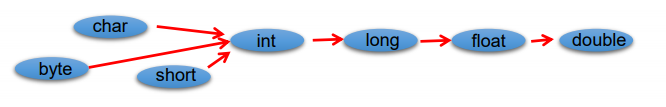将容量大的类型转换成容量小的类型，需要进行强制转换
注意：只要不超出范围可以将整型值直接赋值给 byte，short，char
在多种类型混合运算过程中，首先先将所有数据转换成容量最大的那种，再运算.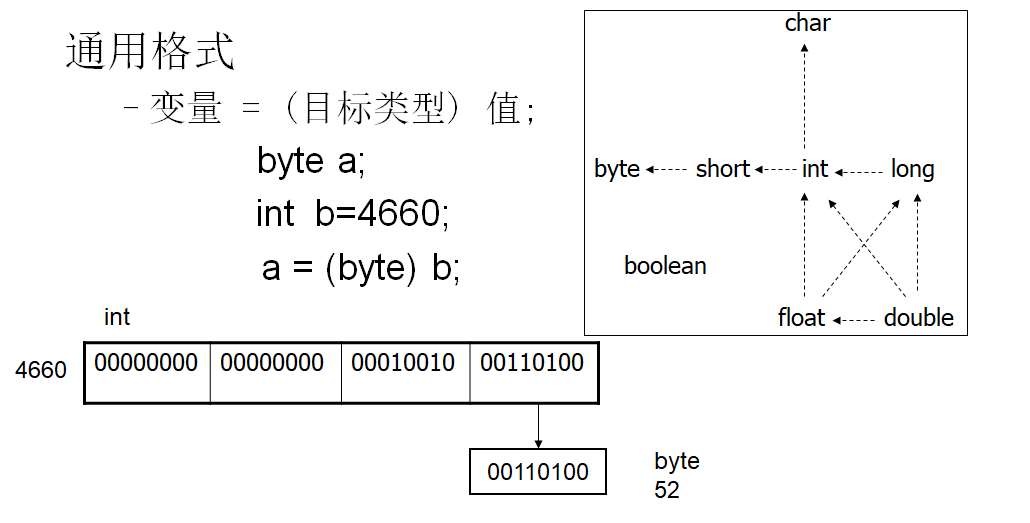有多种类型的数据混合运算时，系统首先自动将所有数据转换成容量最大的 那种数据类型，然后再进行计算。
byte,short,char之间不会相互转换，他们三者在计算时首先转换为int类型。
boolean类型不能与其它数据类型运算。
当把任何基本数据类型的值和字符串(String)进行连接运算时(+)，基本数据类 型的值将自动转化为字符串(String)类型。

五、进制

所有数字在计算机底层都以二进制形式存在。

对于整数，有四种表示方式：

二进制(binary)：0,1 ，满2进1.以0b或0B开头。
十进制(decimal)：0-9 ，满10进1。
八进制(octal)：0-7 ，满8进1. 以数字0开头表示。
十六进制(hex)：0-9及A-F，满16进1. 以0x或0X开头表示。此处的A-F不区分大小写。 如：0x21AF +1= 0X21B0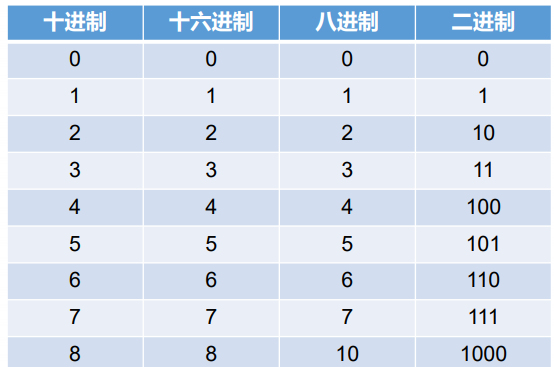1.二进制

Java整数常量默认是int类型，当用二进制定义整数时，其第32位是符号位； 当是long类型时，二进制默认占64位，第64位是符号位

二进制的整数有如下三种形式：

原码：直接将一个数值换成二进制数。最高位是符号位
负数的反码：是对原码按位取反，只是最高位（符号位）确定为1。
负数的补码：其反码加1。

计算机以二进制补码的形式保存所有的整数。

正数的原码、反码、补码都相同
负数的补码是其反码+1

为什么要使用原码、反码、补码表示形式呢？

计算机辨别“符号位”显然会让计算机的基础电路设计变得十分复杂! 于是人们想出了将符号位也参与运算的方法. 我们知道, 根据运算法则减去一个正数等于加上一个负数, 即: 1-1 = 1 + (-1) = 0 , 所以机器可以只有加法而没有减法, 这样计算机运算的设计就更简单了。
2.进制间转化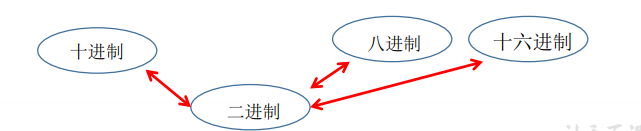十进制 二进制互转

二进制转成十进制 乘以2的幂数
十进制转成二进制 除以2取余数

二进制 八进制互转

二进制 -> 八进制
​	1100 1000   每三位看成一位八进制数 得011  001 000  得 310

二进制 十六进制互转

二进制 ->  十六进制
​    1100 1000   每四位看成一位十六进制数 得 C8

十进制 八进制互转  先转成二进制

十进制 十六进制互转 先转成二进制

六、运算符
​	运算符是一种特殊的符号，用以表示数据的运算、赋值和比较等。

算术运算符
赋值运算符
比较运算符（关系运算符）
逻辑运算符
位运算符
三元运算符

1.算术运算符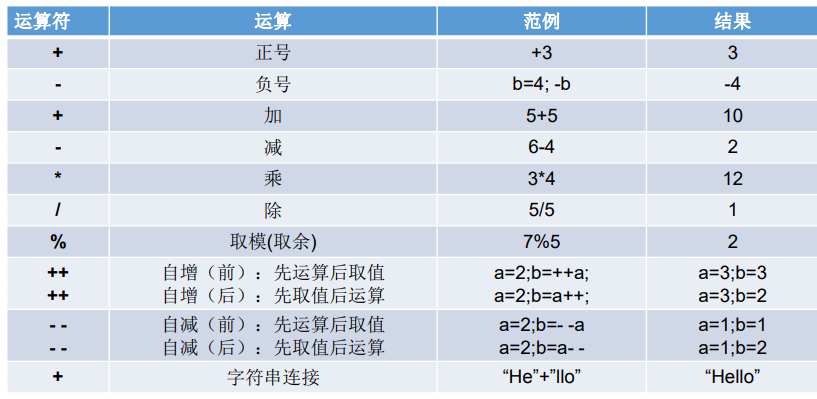算术运算符的注意问题

如果对负数取模，可以把模数负号忽略不记，如：5%-2=1。 但被模数是 负数则不可忽略。此外，取模运算的结果不一定总是整数。
对于除号“/”，它的整数除和小数除是有区别的：整数之间做除法时，只保留整数部分而舍弃小数部分。 例如：int x=3510;x=x/1000*1000; x的结果是？
“+”除字符串相加功能外，还能把非字符串转换成字符串.例如： System.out.println(“5+5=”+5+5); //打印结果是？ 5+5=55 ?

练习1：算术运算符：自加、自减

java
public class SignTest{
public static void main(String[] args){
int i1 = 10;
int i2 = 20;
int i = i1++;
System.out.println("i="+i);
System.out.println("i1="+i1);
i = ++i1;
System.out.println("i="+i);
System.out.println("i1="+i1);
i = i2--;
System.out.println("i="+i);
System.out.println("i2="+i2);
i = --i2;
System.out.println("i="+i);
System.out.println("i2="+i2);
}
}


练习2

随意给出一个整数，打印显示它的个位数，十位数，百位数的值。 格式如下：  数字xxx的情况如下： 个位数： 十位数： 百位数：  例如：  数字153的情况如下：  个位数：3  十位数：5  百位数：1

## **2.赋值运算符**

- 符号：=

- - 当“=”两侧数据类型不一致时，可以使用自动类型转换或使用强制 类型转换原则进行处理。
- 支持连续赋值。

扩展赋值运算符： +=, -=, *=, /=, %=

## **3. 比较运算符**

- 比较运算符的结果都是boolean型，也就是要么是true，要么是false。
- 比较运算符“==”不能误写成“=” 。

思考1：

boolean b1 = false; //区分好==和=的区别。  if(b1==true)     System.out.println("结果为真");  else     System.out.println("结果为假");

## **4 .逻辑运算符**

&—逻辑与  | —逻辑或   ！—逻辑非   && —短路与   || —短路或   ^ —逻辑异或

![$外链图片转存失败,源站可能有防盗链机制,建议将图片保存下来直接上传(img-CS8cBX7j-1573261254860)(G:\ALanQiao\Day03_进制运算符等基础\图片\14.png)$](https://img-blog.csdnimg.cn/20191109091422276.png?x-oss-process=image/watermark,type_ZmFuZ3poZW5naGVpdGk,shadow_10,text_aHR0cHM6Ly9ibG9nLmNzZG4ubmV0L3FxXzQxMjA0OTgz,size_16,color_FFFFFF,t_70)

- 逻辑运算符用于连接布尔型表达式，在Java中不可以写成33 & x<6 。

- “&”和“&&”的区别：

- - 单&时，左边无论真假，右边都进行运算；
- 双&时，如果左边为真，右边参与运算，如果左边为假，那么右边不参与运算。

- “|”和“||”的区别同理，||表示：当左边为真，右边不参与运算。

- 异或( ^ )与或( | )的不同之处是：当左右都为true时，结果为false。

理解：异或，追求的是“异”!

## **5.位运算符**

-  位运算是直接对整数的二进制进行的运算

## **6.三元运算符**

- 格式:
![在这里插入图片描述](https://img-blog.csdnimg.cn/20191109091454813.png)

- 表达式1和表达式2为同种类型
- 三元运算符与if-else的联系与区别：

1）三元运算符可简化if-else语句

2）三元运算符要求必须返回一个结果。

3）if后的代码块可有多个语句

- 练习： 获取两个数中的较大数 获取三个数中的较大数

## **7.运算符的优先级**

- 运算符有不同的优先级，所谓 优先级就是表达式运算中的运 算顺序。如右表，上一行运算 符总优先于下一行。
- 只有单目运算符、三元运算符、 赋值运算符是从右向左运算的。



展开全文• 二、java关键字和保留字 1、关键字 定义：被java语言赋予了特殊涵义的单词。 特点：关键字中所有字母均小写。 关键字共有50个，分为以下类型，都有其特殊含义。 用于定义数据类型的关键字(下表)

一、java语言基础构成
java语言的基础由关键字、标识符、注释、常量和变量、运算符、语句、函数、数组构成。
二、java关键字和保留字
1、关键字
定义：被java语言赋予了特殊涵义的单词。
特点：关键字中所有字母均为小写。
关键字共有50个，分为以下类型，都有其特殊含义。

用于定义数据类型的关键字(下表)

class
interface
byte
short
int
long
float
double
char
boolean
void

用于定义数据类型值的关键字：true，false，null用于定义流程控制的关键字(下表)

if
else
switch
case
default
while
do
for
break
continue
return

用于定义访问权限修饰符的关键字：private， protected，public用于定义类、函数、变量修饰符的关键字：abstract，final，static，synchronized用于定义类与类之间关系的关键字：extends， implements用于建立实例及引用实例，判断实例的关键字：new，this，super，instanceof用于异常处理的关键字：try，catch，finally，throw，throws用于包的关键字：package，import其它修饰符关键字：native，strictfp(strict float point,精确浮点)，                                transient(变量修饰符，用它声明一个实例变量，在对象序列化时不会被序列化)，
volatile(类型修饰符，用来修饰被不同线程访问和修改的变量)，
assert(断言，可以看作是异常处理的一种高级形式，用于捕捉我们在代码中做出的假设)。
2、保留字：以后可能用的。const、goto
三、java中的常量与变量
1、常量
常量：表示不能改变的数值。
java中常量的分类：

整数常量：所有整数小数常量：所有小数布尔型常量：较为特有，只有两个数值，true和false字符常量：将一个数字字母或符号用单引号(‘’)标识字符串常量：将一个或者多个字符用双引号标识null常量：只有一个数值，null
对于整数，java有三种表现形式：

二进制：0和1表示，满2进1十进制：0~9，满10进1八进制：0~7，满8进1，开头用O表示十六进制：0~9，A~F，满16进1，开头用Ox表示计算机采用二进制的原因：

技术实现简单，计算机是由逻辑电路组成，逻辑电路通常只有两个状态，开关的接通与断开，这两种状态正好可以用“1”和“0”来表示。简化运算规则，两个二进制数和、积运算组合各有三种，运算规则简单，有利于简化计算机内部结构，提高运算速度。适合逻辑运算，逻辑代数是逻辑运算的理论依据，二进制只有两个数码，正好与逻辑代数的“真”与“假”相吻合。易于进行转换，二进制与十进制数易于互相转换。用二进制表示数据具有抗干扰能力强，可靠性高等优点。因为每位数据只有高低两个状态，当受到一定程度的干扰时，仍能可靠的分辨出它是高还是低。
2、变量

定义：内存中的一个存储空间，该空间有自己的名称(变量名)和类型(数据类型)。作用：用于不断的存放同一类型的常量，并可以重复使用。定义的格式：数据类型   变量名  = 初始化值。 如：int x = 6;变量的作用范围：从变量定义开始到定义它的代码块结束。同一范围内，不允许多个局部变量命名冲突。什么时候定义变量：当数据不确定的时候，需要对一个数据进行存储时，就定义一个变量来完成存储动作。变量的命名规则：
①应以字母、下划线、或美元符号开头。
②后面接字母、下划线、美元符号或者是数字。
③java变量名没有长度限制。
④java变量名对大小写敏感。
驼峰命名法：
①变量名应该用有意义的单词。
②变量名如果只有一个单词，则所有的字母小写。
③变量名如果由多个英文单词组成，则从第二个单词开始首字母大写。
下面举一些例子来说明命名规则：
合法的变量名：Password、last_name、$abc、_ref 正确的变量名：password、last_Name3、数据类型 java是一种强类型语言。这就意味着java必须为没一个变量声明一种类型。在java中，一共有8中基本类型，其中有4种整型、2种浮点类型、一种用于表示Unicode编码的字符单元的字符类型char和一种用于表示真值的boolean类型。java中有两种基本数据类型：基本数据类型和引用数据类型，其结构图如下：基本数据类型：所占字节数及取值范围如下图：基本数据类型的转换： 在java中基本数据类型共有8中，其中boolean型不能转换成其它类型，其它类型也不能转换呈boolean类型。 除boolean类型外，剩下的7种类型按照他们容量的大小，从小到大，依次排序为： 容量小的向容量大的，可以自动转换，比如可以将一个int类型的值直接赋值给double，如：double d = 34；容量大的向容量小的转换，则必须进行强制类型转换，如：int i = (double)32.42;整数类型常量默认为int类型，浮点型常量默认为double类型在多种数据类型进行混合运算时，容量小的自动转换成整个表达式中容量最大的，然后再进行计算。在进行多种数据类型混合运算时，byte、short、char三种类型不会相互转换，他们各自转换成int再进行计算。在有些时候，容量小的会自动提升为容量大的，比如方法重载传递参数的时候，但byte、short不会自动提升为char。注意：强制类型转换虽然能通过编译，但运行时，有可能会造成数据溢出，损失精度。自动类型提升示意图如下：四、java中的运算符 1、算术运算符 单目运算符：+(正)、-(负)、++(自增)、--(自减)双目运算符：(+、-、*、/、%)(加、减、乘、除、模)三目运算符：a>b?表达式1：表达式2。注：当a>b为true时，返回表达式1的值，否则返回表达式2的值。package com.huang.test; /** *@author huangxiang *@date 创建时间：2015年3月10日下午10:32:30 *@version 1.0 */ public class TestDemo { //演示算术运算符 public static void main(String[] args) { //单目运算符的演示 int a = 5,b=4,c=8; int d = a+b-c*b; int e = a>d?a:d;//三目运算符 System.out.println(d);//d = -23 System.out.println(e);//e = 5; } } 2、赋值运算符：=、+=、-=、*=、/=、%= 3、比较运算符：==(比较的是左右两边的变量是否来自于同一个内存地址) 注：①==运算符比较的是值类型，(如基础数据类型，int之类的)的话，由于值类型是存储在栈里面的，当两个变量有同一个值时，其 实他们只用到了一个内存空间，所以比较的结果是true。 public class Test2Demo { public static void main(String[] args) { int a = 1; int b = 1; System.out.println(a==b);//结果为true } } ②如果比较两个引用类型，则比较的就是它们的引用地址。这时候就要看两个变量是否引用自同一个地址的内存了。 public class Test2Demo { public static void main(String[] args) { String s1 = "abc"; String s2 = "abc"; System.out.println(s1==s2);//结果为true } } 但是如果： public class Test2Demo { public static void main(String[] args) { String s1 = "abc"; String s2 = new String("abc"); System.out.println(s1==s2);//结果为false } }为什么结果会不同呢？ 原因：a、java中有字符串缓冲池。在例子1中，S1和S2用的是同一个内存空间的“abc”，所以此时==的结果为true。 b、在例子2中，S2使用了new操作符重新申请了空间，没有用到缓冲池里的原有变量，因此，虽然S1和S2的值都是"abc"。 但所属不同内存空间，所以==的结果为false。 4、逻辑运算符(&&、||、!、|、&、^) 其中，&和&&的特点是：单&时，左边无论真假，右边都进行运算；双&时，如果左边为真，右边参与运算；如果左边为假，则右边不参与运算。而“|”和“||”的特点是：“|”表示其两边都参与运算。而“||”时，表示当左边为真，右边不参与运算。 5、位运算符(&、|、^、~、>>、<<、>>>) 左移(<<)：被移除的高位丢弃，空位补0右移(>>)：被移位的二进制最高位是0，则右移后空位补0；最高位为1，空位补1。无符号右移(>>>)：被移位的二进制无论最高位是什么，都补0与(&)：与二进制位进行&运算，两个全部为1，结果为1，否则为0或(|)：与二进制为进行|运算，只有两个都为0是才是0，否则为1异或(^)：两个操作数的位中，相同结果为0，不同为1非(~):取反. 6、Instanceof 该运算符是双目运算符，左边操作的是一个对象，右边操作的是一个类或接口。当左面的对象是右边的类(或右边的类的子孙类)创建的对象、或者是右边接口的实现类（或实现的子孙类）的对象时，该运算符结果为true，否则为false。 五、标识符 定义：在java语言中，对于变量、常量、函数、语句块也有名字，我们通通称之为java标识符。标识符是用来给类、对象、方法、变量和自定义数据类型命名的。 标识符的组成：标识符由数字、字母、下划线、美元符号组成。java中是区分大小写的，而且还要求首字母不能是数字。最重要的是，java关键字不能当做标识符。 如： 合法标识符：myName、My_name、Points、_3_ 非法标识符：#name、25name、class、&time 标识符命名约定： 类名和接口：每个字的首字母大写，含有大小写。如;Myclass、HelloWorld等方法名：首字母小写，其余的字母大写，含有大小写，尽量少用下划线。常量名：基本数据类型的常量名全部使用大写字母，字与字之间用 下划线分隔。对象常量可用大小写混写。如：SIZE_NAME。变量名：可大小写混写，首字母小写，字间 分隔符用字的首字母大写。不用下划线，少用美元符号。给变量名命名尽量见名知意。  展开全文语言 • Java-基本语法 关键字和保留字 关键字(keyword)的定义和特点 定义：被Java语言赋予了特殊含义，用做专门用途的字符串（单词） 特点：关键字中所有字母都小写 Java保留字：现有Java版本尚未使用，但以后... 目录 Java-基本语法上 关键字和保留字 标识符(Identifier) 变量 运算符 参考文献 Java-基本语法上 关键字和保留字 关键字(keyword)的定义和特点 定义：被Java语言赋予了特殊含义，用做专门用途的字符串（单词） 特点：关键字中所有字母都为小写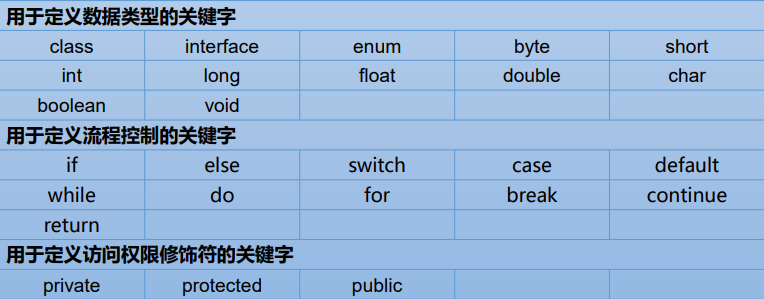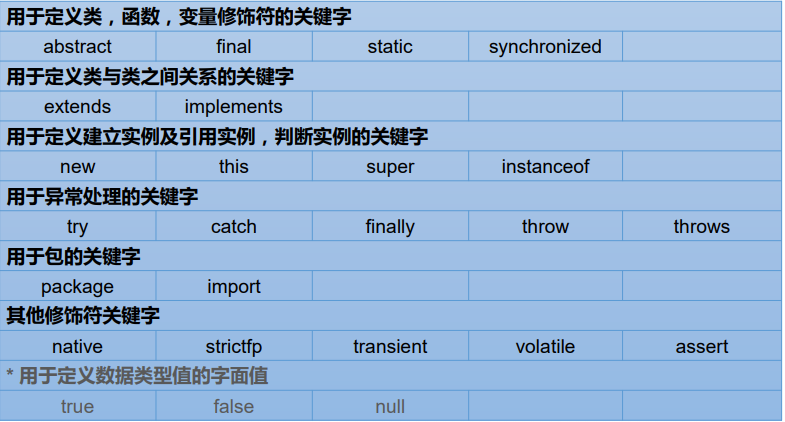Java保留字：现有Java版本尚未使用，但以后版本可能会作为关键字使用。自己命名标识符时要避免使用这些保留字 goto 、const。 标识符(Identifier) 标识符 Java 对各种变量、方法和类等要素命名时使用的字符序列称为标识符 技巧：凡是自己可以起名字的地方都叫标识符。 命名规则：(如果不遵守以下的规则，编译不通过！需要大家严格遵守) 由26个英文字母大小写，0-9 ，_或$ 组成
数字不可以开头。
不可以使用关键字和保留字，但能包含关键字和保留字。
Java中严格区分大小写，长度无限制。
标识符不能包含空格。
Java中的名称命名规范：(如果不遵守以下的规范，编译可以通过！建议大家遵守)

包名：多单词组成时所有字母都小写：xxxyyyzzz
类名、接口名：多单词组成时，所有单词的首字母大写：XxxYyyZzz   大驼峰式
变量名、方法名：多单词组成时，第一个单词首字母小写，第二个单词开始每个 单词首字母大写：xxxYyyZzz   小驼峰式
常量名：所有字母都大写。多单词时每个单词用下划线连接：XXX_YYY_ZZZ
注意一：在起名字时，为了提高阅读性，要尽量有意义，“见名知意”。多积累，多看，多模仿。

注意二：java采用unicode字符集，因此标识符也可以使用汉字声明，但是不建议使用

变量

变量的概念：

内存中的一个存储区域
该区域的数据可以在同一类型范围内不断变化
变量是程序中最基本的存储单元。包含变量类型、变量名和存储的值。
变量的作用：

用于在内存中保存数据
使用变量注意：

Java中每个变量必须先声明，后使用   (强类型语言：需要明确指出变量的类型)
使用变量名来访问这块区域的数据
变量的作用域：其定义所在的一对{ }内
变量只有在其作用域内才有效
同一个作用域内，不能定义重名的变量
class VariableTest{
public static void main(String[] args){
//java定义变量的格式 ： 数据类型 变量名 = 变量值;
int  mgAge = 12;
System.out.println(mgAge);

int myNumber;
myNumber = 1601012;
System.out.println(myNumber);
}
}

变量类型

按数据类型：对于每一种数据都定义了明确的具体数据类型（强类型语言），在内存中分配了不同大小的内存空间。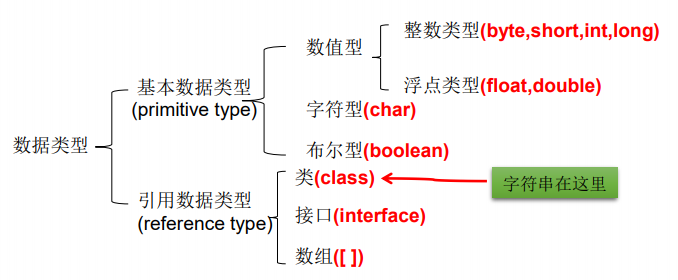按声明的位置的不同

在方法体外，类体内声明的变量称为成员变量
在方法体内部声明的变量称为局部变量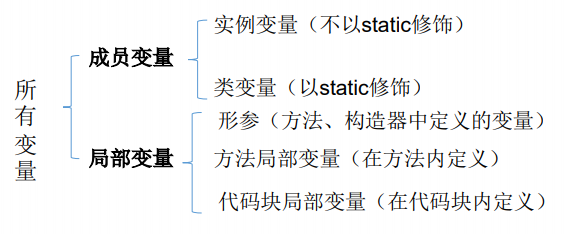各种基本数据类型的使用

package com.atguigu.java;

public class VariableTest1 {
public static void main(String[] args) {
//1.整型 byte(单字节) short(双字节) int(四字节) long(八字节)
//byte 范围 -128 ----  127
byte b1 = 12;
byte b2 = -128;
//b2 = 128; 编译错误
System.out.println(b1);
System.out.println(b2);

//声明long型变量必须以"l"或"L"结尾
//通常定义整型变量时 使用int即可
short s1 = 128;
int i1 = 1234;
long l1 = 1234656l;
System.out.println(l1);

//2.浮点型 float(四字节)  double(八字节)
//浮点型：表示带有小数点的数值
//float表示数值的范围要比long的范围还大

double d1 = 123.3;
System.out.println(d1);

//定义float类型时 变量必须以"f"或"F"结尾
float f1 = 123.2f;
System.out.println(f1);
//通常，定义浮点型变量时 优先使用double

//3.字符型 (双字节)
//定义char型变量时 通常使用一对'',内部只能写一个字符
char c1 = 'a';
//c1 = 'ab';  编译不通过
System.out.println(c1);
//表示方式 1.声明一个字符 2.转义字符 3.直接使用Unicode来表示字符型常量
char c5 = '\n';
c5 = '\t';
System.out.print("hello" + c5);
System.out.println("World");

//4.布尔型 boolean
//只能取两个值之一：ture or false
//常常在条件判断,循环结构中使用
boolean bb1 = true;
System.out.println(bb1);

boolean isMarried = true;
if(isMarried) {
System.out.println("不能参加单身party");
}else {
System.out.println("多找找对象！");
}
}
}


//结果
12
-128
1234656
123.3
123.2
a
hello	World
true
不能参加单身party

基本数据类型之间的运算规则

自动类型转换：容量小的类型自动转换为容量大的数据类型
强制类型转换
package com.atguigu.java;
/*
基本数据类型之间的运算规则：

前提：这里只讨论7种基本数据类型变量间的运算

1.自动类型提升:
当容量小的数据类型的变量与容量大的数据类型的变量作运算时，结果自动提升为容量大的数据类型
byte,char,short --> int --> long --> float --> double
特别的：当 byte,char,short这三种变量的数据做运算时，结果为int型

2.强制类型转换:自动类型提升的逆运算
需要使用强转符 :()
注意点:强制类型转换,可能导致精度损失

说明：此时的容量大小指的是,表示数的范围和大小 例 容量(float) > 容量(long)
*/
public class VariableTest2 {
public static void main(String[] args) {
//自动类型提升
byte b1 = 2;
int i1 = 12;
//byte b2 = b1 + i1  编译不通过
int i2 = b1 + i1;
System.out.println(i2);

float f1 = b1 + i1;
System.out.println(f1);

short s1 = 123;
double d1 = s1;
System.out.println(d1);

//********************
char c1 = 'a';
int i3 = 10;
int i4 = c1 + i3;
System.out.println(i4);

short s2 = 10;
char c2 = 'b';
int i5 = s2 + c2;

byte b2 = 10;
int i6 = b2 + c2;

//强制类型转换
double d11 = 12.3;
int i11 = (int)d11;//截断操作
System.out.println(i11);//存在精度损失

//没有精度损失
long l11 = 123l;
short s22 = (short)l11;
System.out.println(s22);
}
}


#结果
14
14.0
123.0
107
12
123

基本数据类型之间的运算规则的两种特殊情况

package com.atguigu.java;

public class VariableTest3 {
public static void main(String[] args) {
//1.编码情况 long型后丢失 'l'或者'L'
//这种情况下 系统自动默认此变量为int型 ,若超出int型的范围，则报错
long l = 123321;
System.out.println(l);
//long l1 = 1314121323546468; 编译错误 过大的整数

//********
//float型后丢失'f' 默认为double型 此时使用float接受属于强制类型转换,需要使用强转符
//float f1 = 123;

//2.编码情况  对常量
//整型常量：默认类型为int型
//浮点型常量：默认类型为double型

byte b1 = 12;
int i1 = b1 + 10;
System.out.println(i1);

float f2 = 123.2f;
double d1 = f2 + 13;
System.out.println(d1);
};
}

#结果
123321
22
136.1999969482422

基本数据类型运算规则的练习

package com.atguigu.java;

public class VariableTest4 {
public static void main(String[] args) {
//判断是否能通过编译
short s = 5;
s = s - 2; //判断：no 整数常量默认为int型  short与int做运算,结果为int型

byte b = 3;
b = b + 4; //判断：no 整数常量默认为int型  byte与int做运算,结果为int型
b = (byte)(b+4); //判断：yes  整数常量默认为int型  byte与int做运算,结果为int型。
//使用强转符 (byte) 可以用byte型接受

char c = ‘a’;
int i = 5;
float d = .314F;
double result = c+i+d; //判断：yes  自动类型提升,double可以接受char int float型运算的结果

byte b = 5;
short s = 3;
short t = s + b; //判断：no  byte与short做运算,结果为int型
}
}


String类型的使用

package com.atguigu.java;
/*
String类型变量的使用
1.String属于引用数据类型,意为字符串
2.声明String类型变量时,使用一对""
3.String可以和8种基本数据类型变量做运算,且只能是连接"+"运算
4.运算结果仍然是String类型
*/
public class StringTest1 {
public static void main(String[] args) {
String s1 = "Hello world";
System.out.println(s1);

String s2 = "a";
String s3 = "";

int number = 1001;
String numberStr = "学号：";
String info = numberStr + number;
System.out.println(info);

boolean b1 = true;
String info1 = info + b1;
System.out.println(info1);
}
}

//结果
Hello world
学号：1001
学号：1001true

String类型的练习1

package com.atguigu.java;
//关于String类型 的练习题
public class StringExer1 {
public static void main(String[] args) {
//练习1
char c = 'a';//97
int num = 10;
String str = "hello";
System.out.println(c + num + str);//107hello
System.out.println(c + str + num);//ahello10
System.out.println(c + (num + str));//a10hello
System.out.println((c + num) + str);//107hello
System.out.println(str + num + c);//hello10a

//练习2
//控制台输出这种格式  ： *	*
System.out.println("*	*");
System.out.println('*' + '\t' + '*');
System.out.println('*' + "\t" + '*');
System.out.println('*' + '\t' + "*");
System.out.println('*' + ('\t' + "*"));

//注：+ 两端都不是String类型,为加法运算
//   + 两端若存在一着者 为String类型 ,为拼接运算
//   char与char 类型的加法运算 结果为 int型(自动类型提升)
}
}

//结果
107hello
ahello10
a10hello
107hello
hello10a
*	*
93
*	*
51*
*	*

String类型的练习2

package com.atguigu.java;

public class StringExer2 {
public static void main(String[] args) {
String str1 = 4;                     //判断对错：no  字符串必须用双引号标识
String str2 = 3.5f + “”;             //判断str2对错：yes  "3.5"
System.out.println(str2);             //输出：”3.5”
System.out .println(3+4+“Hello!”);     //输出：7Hello!
System.out.println(“Hello!”+3+4);     //输出：Hello!34
System.out.println(‘a’+1+“Hello!”);     //输出：98Hello!
System.out.println(“Hello”+‘a’+1);     //输出：Helloa1
}
}


变量之进制

所有数字在计算机底层都以二进制形式存在
对于整数，有四种表示方式：二进制 八进制 十进制 十六进制
运算符

运算符是一种特殊的符号，用以表示数据的运算、赋值和比较等。

算术运算符的使用

package com.atguigu.java;

/*
运算符之一：算术运算符
+ - + - * / % ++(前)  (后)++   --(前)  (后)-- +
*/
public class AriTest1 {
public static void main(String[] args) {
// 除号:/
int num1 = 12;
int num2 = 5;
int result1 = num1 / num2 ;//int运算结果为int 2
System.out.println(result1);

double result2 = (double)num1 / num2;//double与 int 运算结果为double 2.4
System.out.println(result2);

// %:取余运算
//结果的符号与被模数的符号相同
//开发中，经常使用%来判断能否被除尽
System.out.println("12 % 5 = "+ (12%5));
System.out.println("-12 % 5 = "+ (-12%5));
System.out.println("12 % -5 = "+ (12%-5));
System.out.println("-12 % -5 = "+ (-12%-5));

//运算顺序从右往左
//++(前):  b = ++a;  先自增一,后运算
//(后)++:  b = a++;  先运算,后自增一
int a1 = 10;
int b1 = ++a1;
System.out.println("a1 = " + a1 + ",b1 = " + b1);
int a2 = 10;
int b2 = a2++;
System.out.println("a2 = " + a2 + ",b2 = "+ b2);

//注意点:
short s1 = 10;
//s1 = s1 + 1;//编译错误
//s1 = (short)(s1+1);//编译正确
s1++;//自增一 不会改变本身变量的数据类型
System.out.println(s1);

}
}

//结果
2
2.4
12 % 5 = 2
-12 % 5 = -2
12 % -5 = 2
-12 % -5 = -2
a1 = 11,b1 = 11
a2 = 11,b2 = 10
11

自增自减的练习题

package com.atguigu.java;

public class AriRxer1 {
public static void main(String[] args) {
int i1 = 10;
int i2 = 20;
int i = i1++;//i = 10 i1 = 11
System.out.print("i="+i);
System.out.println("i1="+i1);
i = ++i1;//i1 = 12 i = 12
System.out.print("i="+i);
System.out.println("i1="+i1);
i = i2--;//i = 20 i2 = 19
System.out.print("i="+i);
System.out.println("i2="+i2);
i = --i2;//i2 = 18 i = 18
System.out.print("i="+i);
System.out.println("i2="+i2);
}
}

//结果
i=10i1=11
i=12i1=12
i=20i2=19
i=18i2=18

赋值运算符的使用

package com.atguigu.java;
/*
运算符之二:赋值运算符
= += -= *= /= %=
*/
public class SetVauleTest1 {
public static void main(String[] args) {
//连续赋值
int i1,j1;
i1 = j1 = 10;

int i2 = 10,j2 = 10;

//扩展运算符
int num1 = 10;
num1 += 2;//num1 = num1 + 2;
System.out.println(num1);//12

short s1 = 12;
s1 += 2;//不会改变变量本身的数据类型
System.out.println(s1);

//开发中 如果希望变量实现自增2的操作 int num = 10;
//一:num = num + 2;
//二:num += 2;推荐

//开发中 如果希望变量实现自增1的操作 int num = 10;
//一:num = num + 2;
//二:num += 2;推荐中
//三:num++;

}
}
//结果
12
14


赋值运算符的练习题

package com.atguigu.java;

public class SetValueExer1 {
public static void main(String[] args) {
short s = 3;
//s = s+2;  编译不通过
s += 2;  //正确

int i = 1;
i *= 0.1;//i = i * 0.1
System.out.println(i);//0  不改变数据类型 int(0.1) = 0
i++;
System.out.println(i);//1

int m = 2;
int n = 3;
n *= m++;// n = n * m++;
System.out.println("m=" + m);//3
System.out.println("n=" + n);//6

int n1 = 10;
n1 += (n1++) + (++n1);//n1 = n1 + (n1++) + (++n1) n1 = 10 + 10 + 12
System.out.println(n1);//32
}
}

比较运算符

package com.atguigu.java;
/*
运算符之三:比较运算符
== != > < >= <= instanceof

结论:
1.比较运算符的结果是boolean类型
2.区分 == 和 =
*/
public class CompareTest {
public static void main(String[] args) {
int i = 10,j = 20;

System.out.println(i == j);//false
System.out.println(i = j);// 20

boolean b1 = true;
boolean b2 = false;
System.out.println(b1 == b2);//true
System.out.println(b1 = b2);//false

}
}

逻辑运算符

package com.atguigu.java;
/*
运算符之四：逻辑运算符
& && | || ! ^

说明:
1.逻辑运算符操作的都是boolean类型的变量
*/
public class LogicTest {
public static void main(String[] args) {
//区分 & 与 &&
//相同点1：  &与 && 运算结果相同
//相同点2：当符号左边是true时,二者都会执行符号右边的操作
//不同点：当符号左边是false时,&会继续执行符号右边的操作,&&不在执行符号右边的操作
// 开发中推荐使用 && ||
boolean b1 = false;
int num1 = 10;
if(b1 & (num1++ >0)) {
System.out.println("我现在在北京");
}else {
System.out.println("我现在在南京");
}//我现在在南京
System.out.println("num1 = " + num1);//num1 = 11

boolean b2 = false;
int num2 = 10;
if(b2 && (num2++ >0)) {
System.out.println("我现在在北京");
}else {
System.out.println("我现在在南京");
}//我现在在南京
System.out.println("num2 = " + num2);//num2 = 10

//区分 | 与 ||
//相同点1： | 与 || 运算结果相同
//相同点2：当符号左边是false时,二者都会执行符号右边的操作
//不同点：当符号左边是true时,|会继续执行符号右边的操作,||不在执行符号右边的操作

boolean b3 = true;
int num3 = 10;
if(b3 | (num3++ >0)) {
System.out.println("我现在在北京");
}else {
System.out.println("我现在在南京");
}//我现在在北京
System.out.println("num3 = " + num3);//num3 = 11

boolean b4 = true;
int num4 = 10;
if(b4 || (num4++ >0)) {
System.out.println("我现在在北京");
}else {
System.out.println("我现在在南京");
}//我现在在北京
System.out.println("num4 = " + num4);//num4 = 10
}
}

逻辑操作符的练习

package com.atguigu.java;

public class LogicExer1 {
public static void main(String[] args) {
int x = 1;
int y=1;
if(x++==2 & ++y==2){
x =7;
}
System.out.println("x="+x+",y="+y);//x=2 y=2
//解析 1.x++==2   先运算 (x=1) ==2 false  后自增 x = 2
//2. &运算 前者为false 需要继续看后者
//3.++y==2  先 自增 y = 2 后运算(y=2)==2 true
//4.条件为false 不执行if语句 所以 x=2 y=2

int x = 1,y = 1;
if(x++==2 && ++y==2){
x =7;
}
System.out.println("x="+x+",y="+y);//x=2 y=1
//解析1.x++==2   先运算 (x=1) ==2 false  后自增 x = 2
//2. &&运算 前者为false 不需要看后者 此时y=1
//3.条件为false 不执行if语句 所以 x=2 y=1

int x = 1,y = 1;
if(x++==1 | ++y==1){
x =7;
}
System.out.println("x="+x+",y="+y);//x=7,y=2
//解析:1.x++==1 先运算(x=1)==1 true 后自增 x=2
//2.|运算 前者为true 还需要执行后者
//3.++y==1 先自增 y=2 在运算(y=2)==1 false
//4.条件为true 执行if语句 所以 x=7,y=2

int x = 1,y = 1;
if(x++==1 || ++y==1){
x =7;
}
System.out.println("x="+x+",y="+y);//x=7,y=1
解析:1.x++==1 先运算(x=1)==1 true 后自增 x=2
//2.||运算 前者为true 不需要执行后者 此时y=1
//3.条件为true 执行if语句 所以 x=7,y=1
}
}

package com.atguigu.java;

public class LogicExer2 {
public static void main(String[] args) {
boolean x =true;
boolean y =false;
short z=42;
if((z++==42)&&(y=true)) z++;
if((x = false) || (++z==45)) z++;

System. out.println("z="+z);//z=46
//解析
//1. 赋值 x,y,z
//2 if((z++==42)&&(y=true)) z++;
//2.1 (z++==42)  先运算 (z=42)==42 true 后自增 z = 43
//2.2 &&运算 前者为 true 还需要执行后者
//2.3 (y=true) 将true赋值给y 并判断y是否等于true  结果为true
//2.4条件整体为true 执行 z++; 结果为z = 44
//3 f((x = false) || (++z==45)) z++;
//3.1 (x = false) 结果为false
//3.2 ||运算 前者为false 还需要执行后者
//3.3 (++z==45) 先自增z=45 后运算(z=45)==45 结果为true
//3.4 条件整体为true 执行 z++; 结果为z = 46
//4 输出结果 z=46
}
}

位运算符(有点麻烦 不记了)

三元运算符

package com.atguigu.java;
/*
运算符之六：三元运算符
1.结构 : (条件表达式)?表达式1:表达式2
2.说明
2.1 条件表达式的结果为boolean类型
2.2 根据条件表达式真或假,决定执行表达式1,还是表达式2
如果表达式为true,则执行表达式1
如果表达式为false,则执行表达式2
2.3.表达式1 和 表达式2 要求是一致的
2.4.三元运算符是可以相互嵌套的
3.凡是可以使用三元运算符的地方,都可以改写为if-else.反之,不成立
4.如果程序即可以使用三元运算符,又可以使用if-else结构.那么优先使用三元运算符。原件：简单 高效
if(条件表达式){
表达式1
}else{
表达式2
}
*/
public class SanYuanTest {
public static void main(String[] args) {
//获取两个整数中较大值
int m = 25,n = 12;

int max = (m > n)? m:n;
System.out.println(max);//25

String maxStr = (m > n)?"m大":((m == n)?"m等于n":"m小");
System.out.println(maxStr);// m大

//获取三个数的最大值
int n1 = 12,n2 = 30,n3 = -43;
int max1 = (n1 > n2)?n1:n2;
int max2 = (max1 > n3)?max1:n3;
System.out.println(max2);//30
//不建议这样写
int max3 = (n1 > n2)?((n1 > n3)?n1:n3):((n2>n3)?n2:n3);
System.out.println(max3);//30

}
}

参考文献

B站尚硅谷Java零基础教程-java入门必备_宋红康

https://www.bilibili.com/video/BV1Kb411W75N?
`
展开全文编程语言
• Java中的关键字要注意以下几点1)Java语言中的关键字均小写字母表示。TRUE、NULL等不是关键字。2)goto和const虽然在Java中没有作用，但仍保留作为Java的关键字。分隔符 圆点“.”、分号“；...
• 1.标识符在Java中，变量、常量、函数、类都可以称为程序实体，而他们的名称统称标识符。(1)标识符的命名规则标识符可以由字母、数字、下划线(_)和\$组成，不能以数字开头。标识符严格区分大小写。标识符不能是Java...
• JavaSE：Java基本语法（上）基本语法思维导图关键字和保留字标识符变量基本数据类型使用说明基本数据类型运算引用数据类型使用说明Stiring类型的使用进制说明运算符算术运算符赋值运算符比较运算符逻辑运算符位...编程语言 javase
• 好了言归正传这个网站之所以好，是因为他的条理性，他从简入深的大家讲解有关java教程的相关知识，以下是相关的目录：   java基础教程 java基础 java简介 java环境搭建 java基本语法 java保留java变量 java...
• 这5种元素有着不同的语法含义和组成规则，它们相互配合，共同完成Java语言的语义表达。 Java中的关键字要注意以下几点 1）Java语言中的关键字均小写字母表示。TRUE、NULL等不是关键字。 2）goto和const虽然在...
• java中给方法，变量，常量，以及一些类起名字的标识称之标识符。标识符主要需要注意的有以下几个方面。 不能以数字开头。 以字母下划线，美元符开头，中间不要有空格，没有长度限制，但最好见文识意。 不能与...
• 保留词管理：添加保留词、保留词数据维护 邀请码管理：生成邀请码、邀请码数据维护 广告管理：侧边栏独占展位维护 其他管理：发帖/回帖开关；用户开放/关闭/邀请码注册开关 开放的内容 API Sym 提供了 API ...开源项目
• 符号保留指令是一个Java文档注释指令，可以插入在类和类成员的文档注释中，例如： /** * This class should preserve. * @preserve */ public class Foo { /** * You can specify which field should be ...编译 反编译
• 符号保留指令是一个Java文档注释指令，可以插入在类和类成员的文档注释中，例如： 1 /** 2 * This class should preserve. 3 * @preserve 4 */ 5 public class Foo { 6 /** 7 * You can specify which field ...jocky
• 符号保留指令是一个Java文档注释指令，可以插入在类和类成员的文档注释中，例如： /** * This class should preserve. * @preserve */ public class Foo { /** * You can specify which field should be...
• 2．设 i、j int型变量名，a int型数组名，以下选项中，正确的赋值语句是( )。 A) i = i + 2 B) a = 7; C) i++ - --j; D) a(0) = 66; 3．Java语言的类间的继承关系是( )。 A) 多重的 B) 单重的 C) 线程的 D) ...
• 件以.java为扩展名。 6、从1.3版开始生成的POJO代码目录中可自动添加一个名pojo.ntf.xml的POJO映射通 知档，其中，ID列名默认使用主键名称（若复合主键则采用次序排首位的主键列名） ，而Oracle环境下的...
• 们之前的学习的C语言、Java语言一样同属于C语言语法系列,有很多相似的地方,同时也 有一些不同的地方,因此我们在学习的时候需要注意它们之间的区别,不要混淆。在本章中, 我们需要掌握c#中的变量、数据类型、运算符、...
• 说明: 为以下各项指定命名约定, 包括日期和星期的编号, 默认日期格式, 默认小数点字符和组分隔符, 以及默认的 ISO 和本地货币符号。可支持的区域包括美国, 法国和日本。有关所有区域的信息, 请参阅 Oracle8i ...
• 例如，往表 MyClass中插入二条记录, 这二条记录表示：编号的名Tom的成绩.45, 编号 的名Joan 的成绩.99，编号 的名Wang 的成绩.5. mysql>insert into MyClass values(1,’Tom’,96.45),(2,’Joan...MySQL
• Array的配置项目没有上面介绍的那么直观,默认情况下DWR装载所有的基本类型和可装载的对象,这些包括String,Date等先前介绍的类型.java高级程序员可能会理解什么match的这行有点奇怪. [Z"/> [B"/> [S"/> [I"/> [J"/>...ajax 框架
• Microsoft给C++添加了许多Microsoft专用的关键字，并把Visual Basic演变Visual Basic 2013，保留了一些基本的Visual Basic语法，但在设计上完全不同于原始Visual Basic，从实际应用的角度来看，Visual Basic 2013...# 以下为java语法保留java 订阅